# Essence! Configuration skills of 12 pandas

2022-02-01 21:33:39 PI dada

official account ： Youer cottage
author ：Peter
edit ：Peter

Hello everyone , I am a Peter~

stay Pandas In the course of using , In addition to data , We deal more with forms . In order to better display a tabular data , There must be good settings in the early stage .

This article introduces Pandas Common configuration skills , Mainly based on options and setings To unfold . Push the official website learning address ：pandas.pydata.org/pandas-docs…## Import

This is a way of introducing international practice ！

``````import pandas as pd
Copy code ``````

## Ignore the warning

Because the version is updated , Probably Pandas Some uses of will be removed soon , There are often warnings （ It's not a mistake ）, With the following code, you can ignore the relevant warnings ：

``````#  Ignore the warning
import warnings
warnings.filterwarnings('ignore')
Copy code ``````

## float Type data accuracy

### View default precision

The default is to keep 6 Decimal place . Print the current accuracy in the following way ：

``````pd.get_option( 'display.precision')
Copy code ``````
``````6
Copy code ``````

### Modify precision

Set the precision to 2 position

``````pd.set_option( 'display.precision',2)
#  How to write it 2：pd.options.display.precision = 2
Copy code ``````

Then we print again, and the current accuracy becomes 2 position ：

``````pd.get_option( 'display.precision')
Copy code ``````
``````2
Copy code ``````

## Show rows

### View the number of rows displayed

The default number of rows displayed is 60

``````pd.get_option("display.max_rows")  #  The default is 60
Copy code ``````
``````60
Copy code ``````

The default minimum number of rows is 10 position ：

``````pd.get_option("display.min_rows")  #  Show at least rows
Copy code ``````
``````10
Copy code ``````

### Modify the number of display lines

Modify the maximum number of display lines to 999, Then look at ：

``````pd.set_option("display.max_rows",999)  #  Display the maximum number of lines
Copy code ``````
``````pd.get_option("display.max_rows")
Copy code ``````
``````999
Copy code ``````

Modify the minimum number of lines displayed ：

``````pd.set_option("display.min_rows",20)
Copy code ``````
``````pd.get_option("display.min_rows")
Copy code ``````
``````20
Copy code ``````

### Reset function

Use reset reset_option After the method , The setting will become the default form （ The number ）：

``````pd.reset_option("display.max_rows")
Copy code ``````
``````pd.get_option("display.max_rows")  #  It's back to 60
Copy code ``````
``````60
Copy code ``````
``````pd.reset_option("display.min_rows")
Copy code ``````
``````pd.get_option("display.min_rows")  #  It's back to 10
Copy code ``````
``````10
Copy code ``````

### Regular functions

If we have more than one options Modified settings , If you want to recover at the same time , Using regular expressions, you can reset multiple option.

Here it means with displacy Reset all the settings at the beginning ：

``````# ^ Indicates starting with a character , Here it means with display Start resetting all
pd.reset_option("^display")
Copy code ``````

### Reset all

If you use all, It means to reset all settings ：

``````pd.reset_option('all')
Copy code ``````

## Show Columns

Since you can control the number of lines displayed , Of course, you can also control the number of columns displayed

### View the number of columns displayed

The number of columns displayed by default is 20：

``````pd.get_option('display.max_columns')

#  Another way of writing ： By attributes
pd.options.display.max_columns
Copy code ``````
``````20
Copy code ``````

### Change the number of columns

Modify the number of columns displayed into 100：

``````#  Modified into 100
pd.set_option('display.max_columns',100)
Copy code ``````

View the number of modified Columns ：

``````#  View the modified value
pd.get_option('display.max_columns')
Copy code ``````
``````100
Copy code ``````

### Show all columns

If I set it to None, It means that all columns are displayed ：

``````pd.set_option('display.max_columns',None)
Copy code ``````

### Reset

``````pd.reset_option('display.max_columns')
Copy code ``````

## Change column width

The above is to view the number of columns , The following is to set the width of each column . Single column data width , In the number of characters , Use an ellipsis to indicate when it exceeds .

### Default column width

The default column width is 50 The width of characters ：

``````pd.get_option ('display.max_colwidth')
Copy code ``````
``````50
Copy code ``````

### Change column width

Modify the displayed column width to 100：

``````#  Modified into 100
pd.set_option ('display.max_colwidth', 100)
Copy code ``````

View the displayed column width and length ：

``````pd.get_option ('display.max_colwidth')
Copy code ``````
``````100
Copy code ``````

### Show all columns

Show all columns ：

``````pd.set_option ('display.max_colwidth', None)
Copy code ``````

## Folding function

When we output data width , When the set width is exceeded , Do you want to collapse . Usually use False Do not fold , contrary True To fold .

``````pd.set_option("expand_frame_repr", True)  #  Fold
Copy code ``````
``````pd.set_option("expand_frame_repr", False)  #  Do not fold
Copy code ``````

## Code snippet modification settings

Various settings described above , If there is any modification, it is the of the whole environment ; We can also make temporary settings for only one code block .

Run out of the current code block , It will fail , Restore to the original settings .

Suppose this is the first code block ：

``````print(pd.get_option("display.max_rows"))
print(pd.get_option("display.max_columns"))
60
20
Copy code ``````

Here is the second code block ：

``````#  Set the current code block

with pd.option_context("display.max_rows", 20, "display.max_columns", 10):
print(pd.get_option("display.max_rows"))
print(pd.get_option("display.max_columns"))
20
10
Copy code ``````

Here's the third code block ：

``````print(pd.get_option("display.max_rows"))
print(pd.get_option("display.max_columns"))
60
20
Copy code ``````

In the above example, we can find that ： Outside the specified code block , Invalid settings

## Number formatting

Pandas There was a display.float_format Methods , It can format and output floating-point numbers , For example, use the thousandth , percentage , Fixed decimal places, etc .

If other data types can be converted to floating point numbers , You can also use this method .

The callable should accept a floating point number and return a string with the desired format of the number

### The thousandth represents

When the data is big , Hope to pass Thousandths To represent data , Be clear at a glance ：

``````df = pd.DataFrame({
"percent":[12.98, 6.13, 7.4],
"number":[1000000.3183,2000000.4578,3000000.2991]})
df
Copy code ``````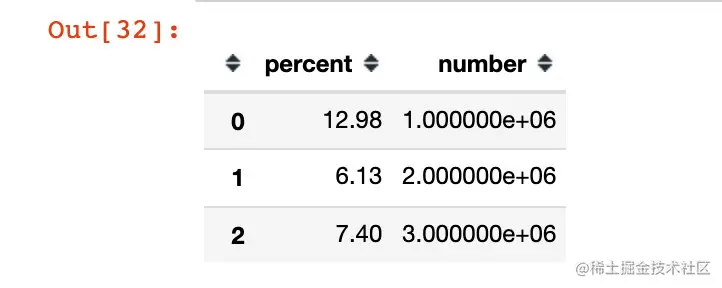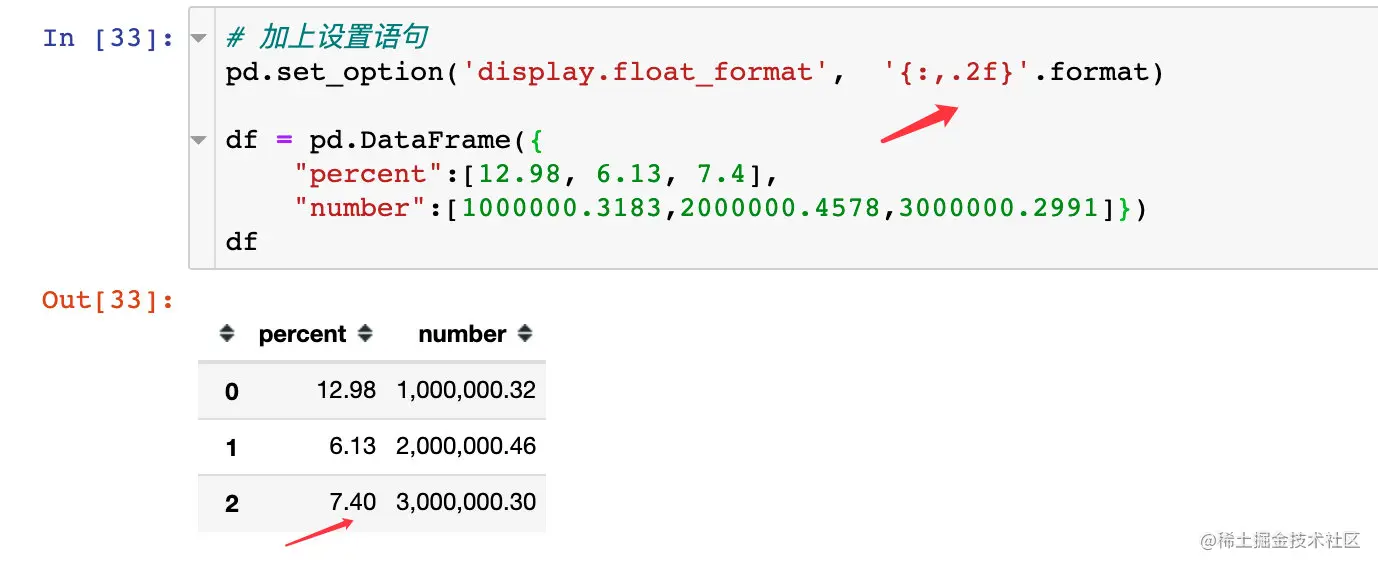### percentage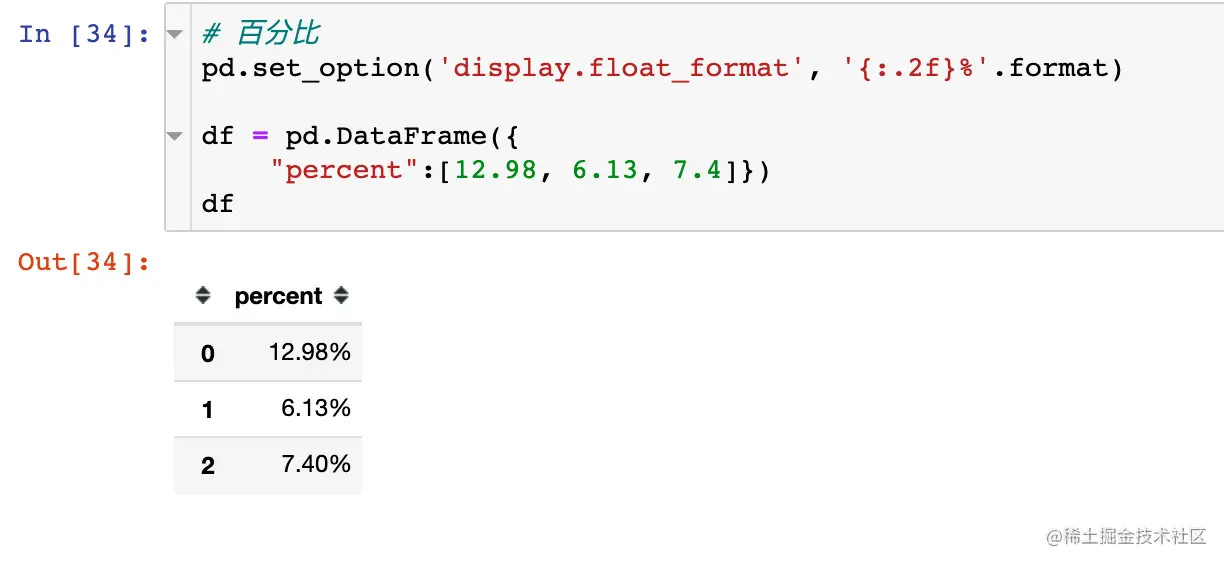### Special symbols

except % Number , We can also use other special symbols to represent ：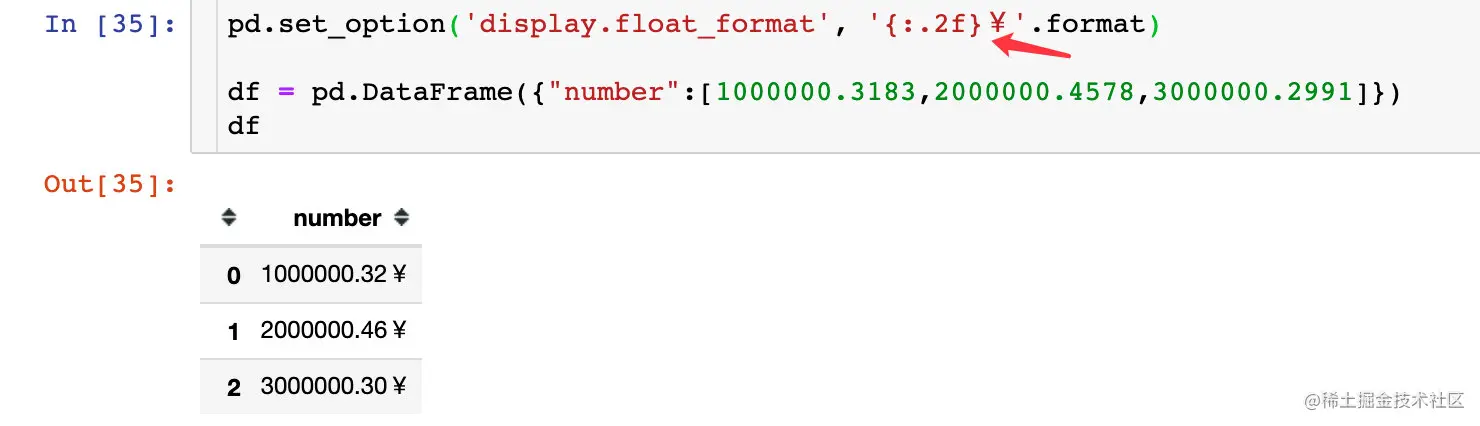## Zero threshold conversion

What does threshold switching mean ？ First of all, the implementation of this function uses display.chop_threshold Method .

It means that you will Series perhaps DF The data in is displayed as the threshold of a certain number . Greater than this number , Direct display ; Less than , use 0 Show .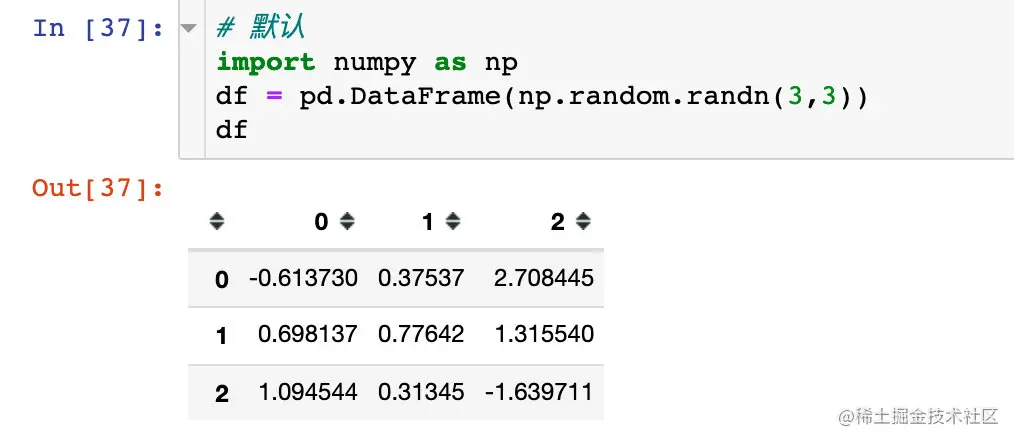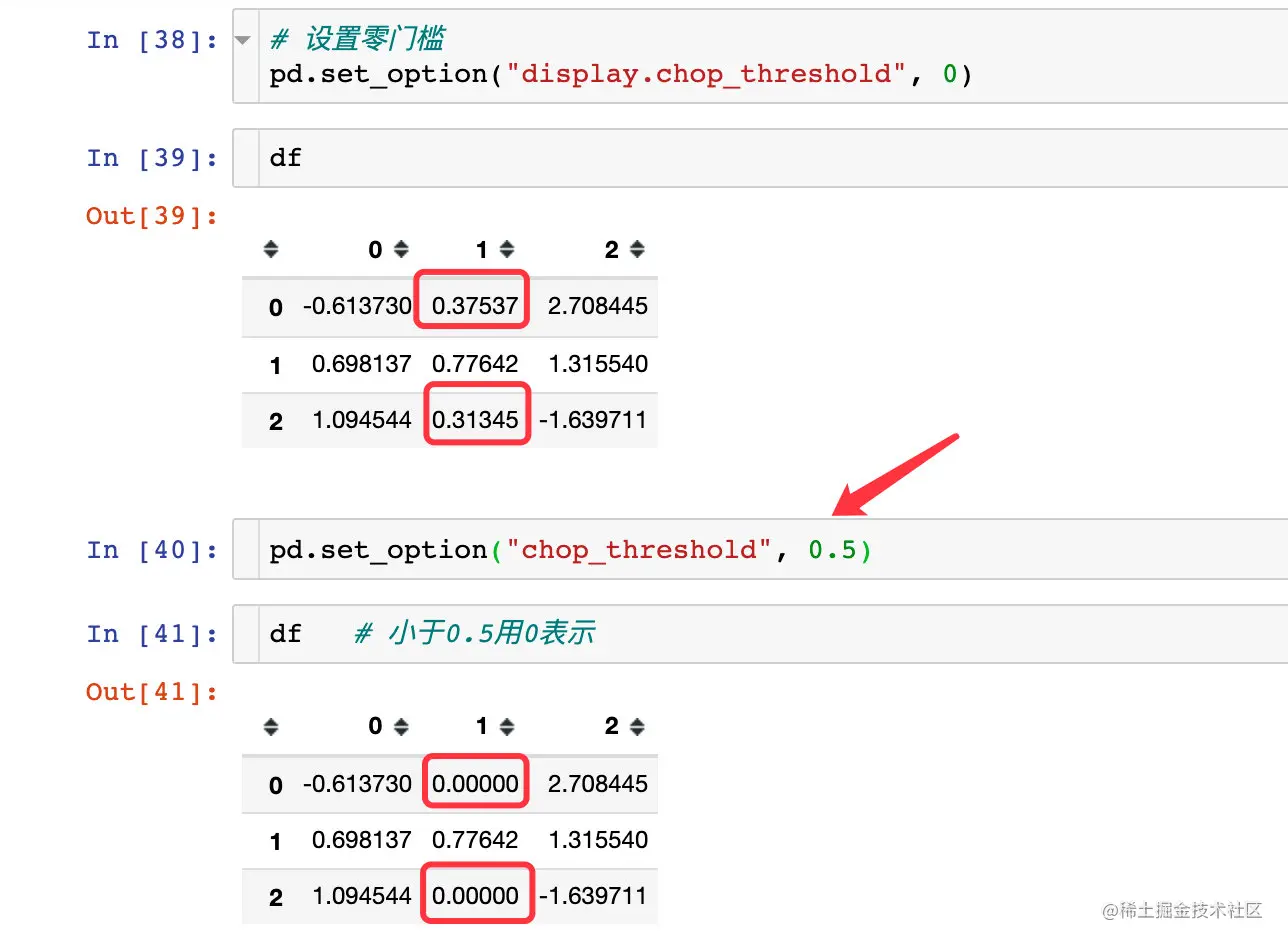## Change the drawing method

By default ,pandas Use matplotlib As drawing backend , We can modify the settings ：

``````import matplotlib.pyplot as plt
%matplotlib inline

#  By default
df1 = pd.DataFrame(dict(a=[5,3,2], b=[3,4,1]))
df1.plot(kind="bar")
plt.show()
Copy code ``````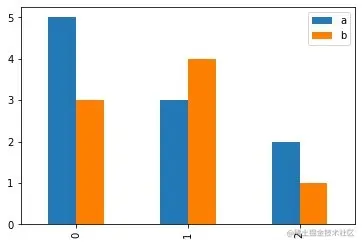Change the back end of the next drawing , Become powerful plotly：

``````#  How to write it 1
pd.options.plotting.backend = "plotly"

df = pd.DataFrame(dict(a=[5,3,2], b=[3,4,1]))
fig = df.plot()
fig.show()

#  How to write it 2
df = pd.DataFrame(dict(a=[5,3,2], b=[3,4,1]))
fig = df.plot(backend='plotly') #  Specify here
fig.show()
Copy code ``````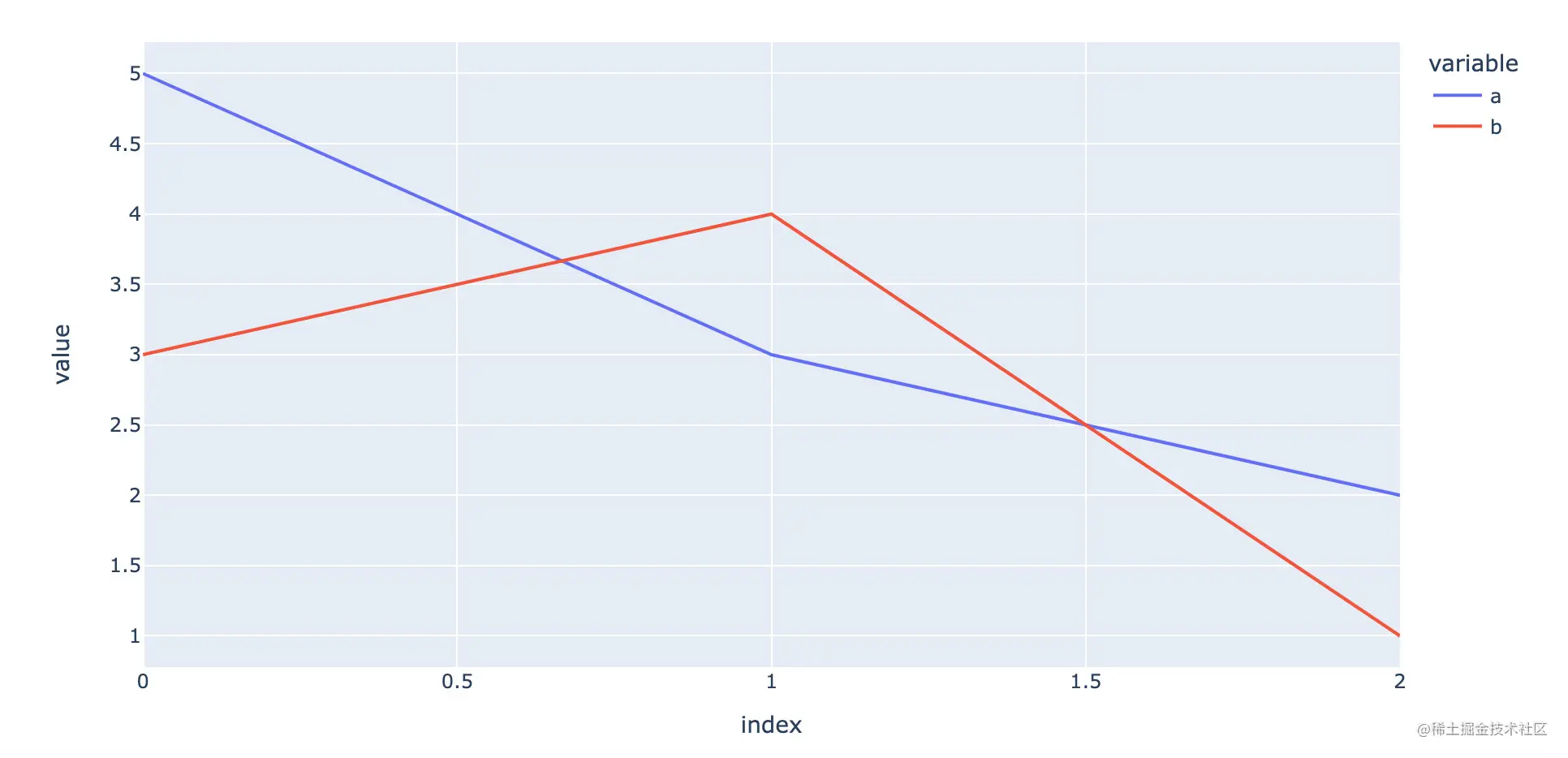## Change the column head alignment direction

By default , attribute field （ The column header ） It's right aligned , We can set . Let's take a look at an example from the official website ：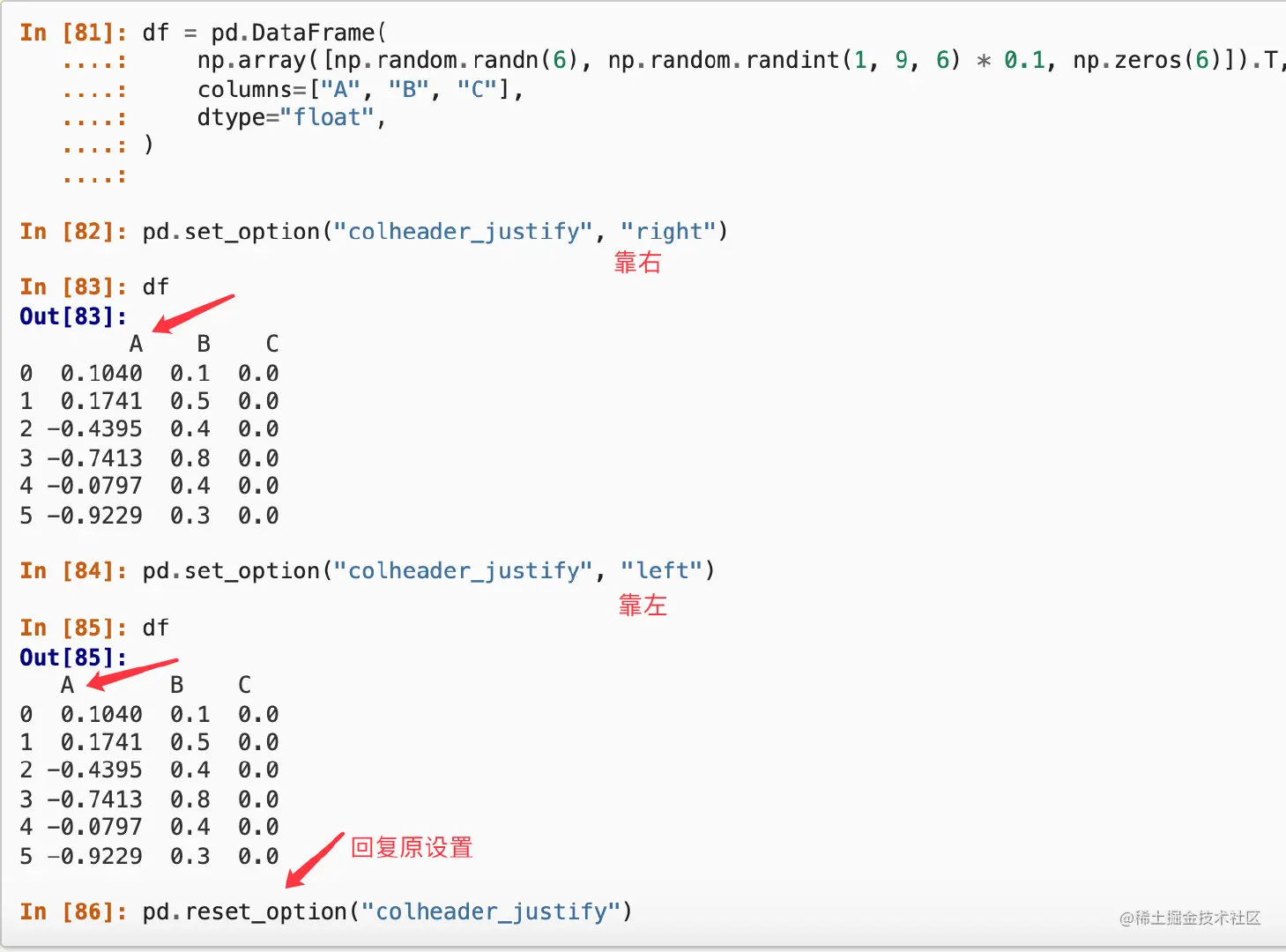## Print out the current settings and reset all options

`pd.describe_option()` Is to print all the current settings , And recharge all options . Here are some setting options ：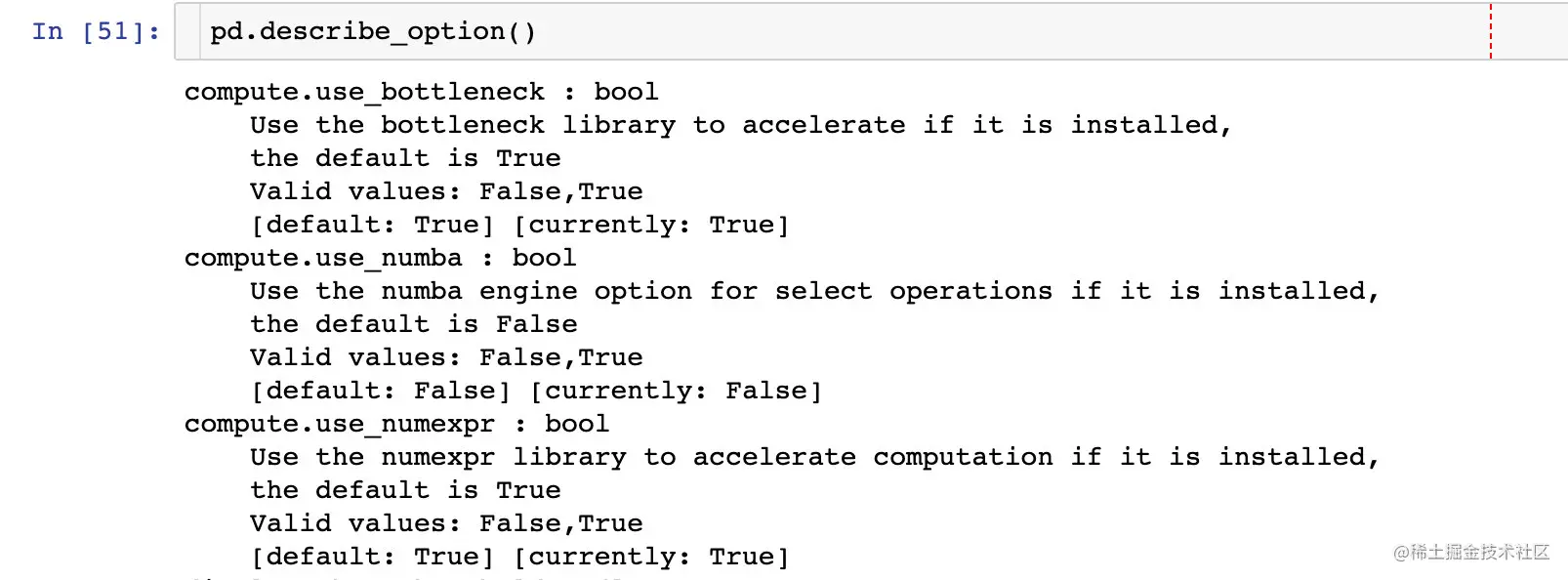## Configuration skills

Common configurations are summarized below , Copy and use ：

``````import pandas as pd  #  International practice

import warnings
warnings.filterwarnings('ignore')  #  Ignore the warning in the text

pd.set_option( 'display.precision',2)
pd.set_option("display.max_rows",999)  #  Display the maximum number of lines
pd.set_option("display.min_rows",20)   #  Minimum number of lines displayed
pd.set_option('display.max_columns',None)  #  All columns
pd.set_option ('display.max_colwidth', 100)   #  Change column width
pd.set_option("expand_frame_repr", True)  #  Fold
pd.set_option('display.float_format',  '{:,.2f}'.format)  #  Thousandths
pd.set_option('display.float_format', '{:.2f}%'.format)  #  Percentage form
pd.set_option('display.float_format', '{:.2f}￥'.format)  #  Special symbols
pd.options.plotting.backend = "plotly"  #  Modify drawing
pd.set_option("colheader_justify","left")  #  Column field alignment
pd.reset_option('all')  #  Reset
Copy code ``````